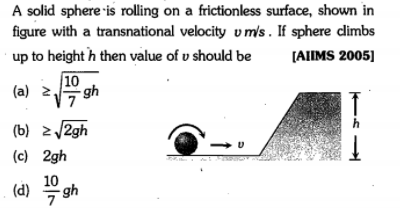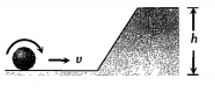Question 23 of 35A solid sphere is rolling on a frictionless surface, shown in figure with a transnational velocity v m/s. If sphere climbs up to height h then value of v should be(a) $\ge \sqrt{\frac{10}{7}\mathrm{gh}}$                                                    (b) $\ge \sqrt{2\mathrm{gh}}$

(c) $2\mathrm{gh}$                                                              (d) $\frac{10}{7}\mathrm{gh}$